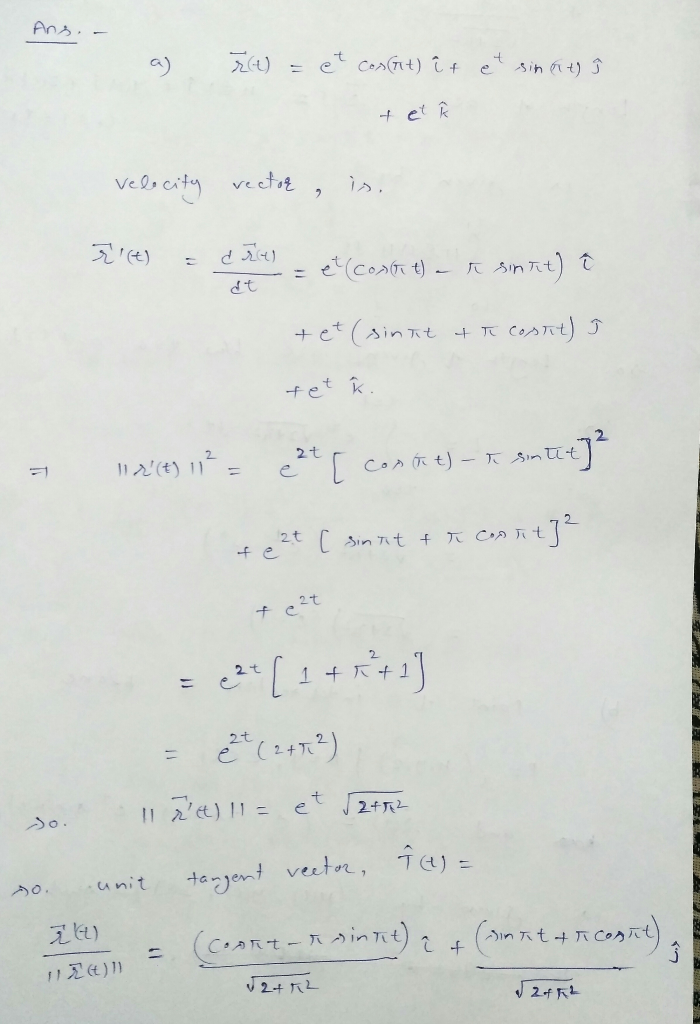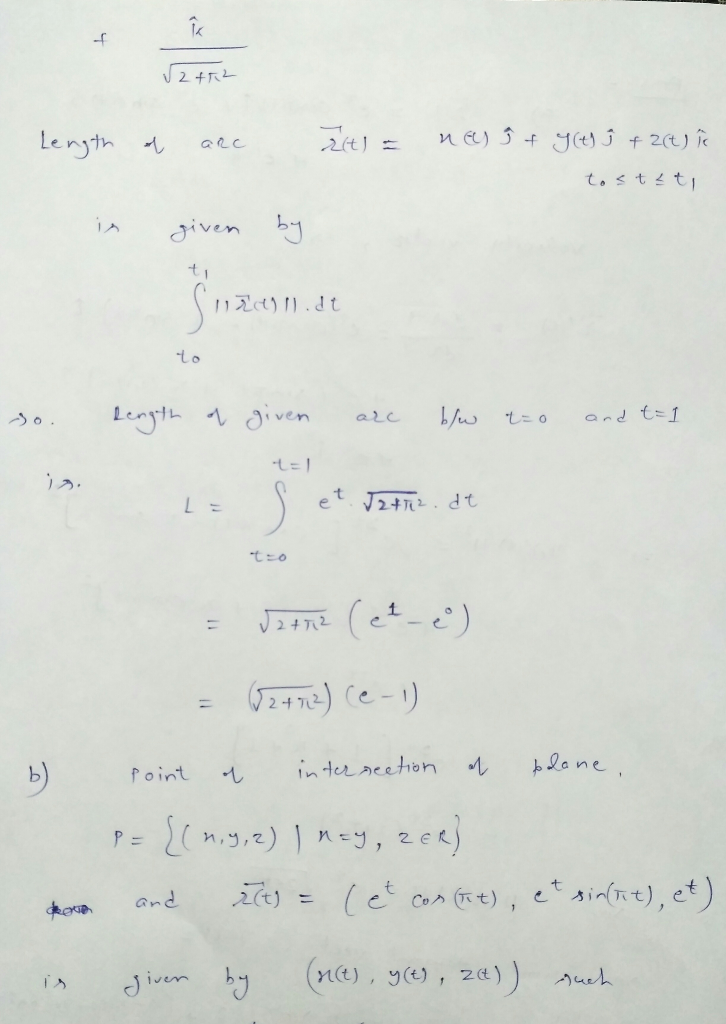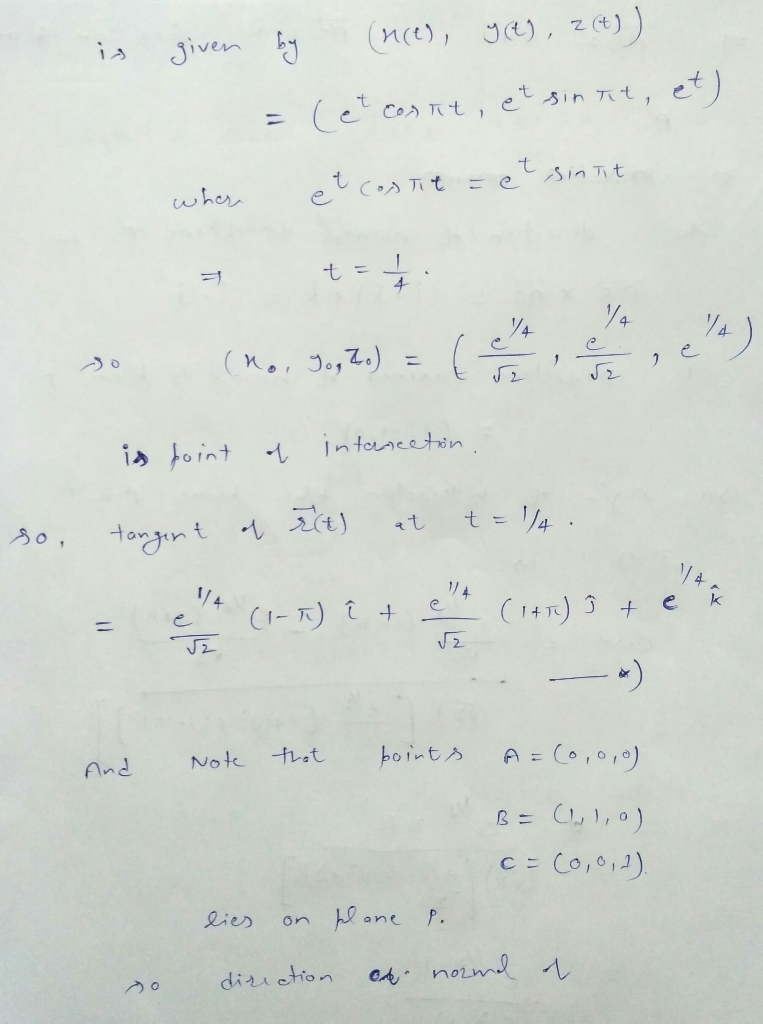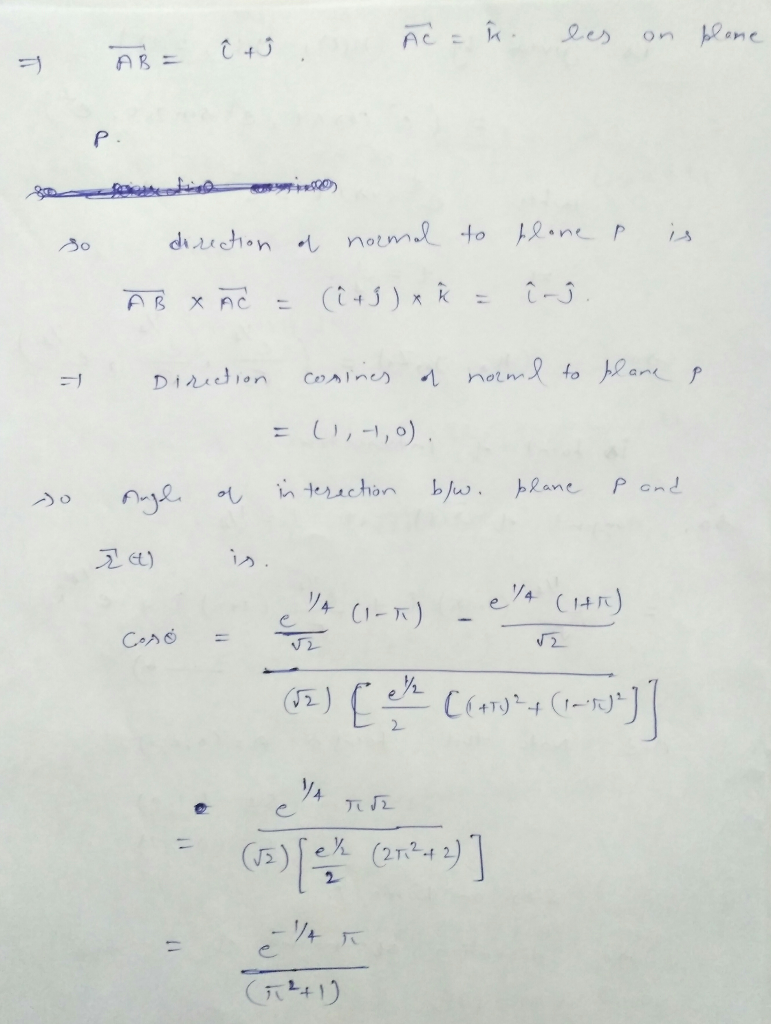# FOR THE PARAMETRIZED PATH r(t)= e^tcos(πt)i+e^tsin(πt)j+e^tk a) find the velocity vector, the unit tangent vector and...

FOR THE PARAMETRIZED PATH r(t)= e^tcos(πt)i+e^tsin(πt)j+e^tk

a) find the velocity vector, the unit tangent vector and the arc lenght between t=0 and t=1

b) find a point where the path given by r(t) intersects the plane x-y=0 and determine the angle of intersection between the tangent vector to the curve and the normal vector to the plane.

## Solutions

##### Expert Solution## Related Solutions

##### Find the point of intersection of the tangent lines to the curve r(t) = 5 sin(πt),...
Find the point of intersection of the tangent lines to the curve r(t) = 5 sin(πt), 2 sin(πt), 6 cos(πt) at the points where t = 0 and t = 0.5. (x, y, z) =
##### 15. a. Find the unit tangent vector T(1) at time t=1 for the space curve r(t)=〈t3...
15. a. Find the unit tangent vector T(1) at time t=1 for the space curve r(t)=〈t3 +3t, t2 +1, 3t+4〉. b. Compute the length of the space curve r(t) = 〈sin t, t, cos t〉 with 0 ≤ t ≤ 6.
##### For this parametrized curve: x = e^(2t) sin t , y = cos(4t) find tangent line...
For this parametrized curve: x = e^(2t) sin t , y = cos(4t) find tangent line to curve when t=1
##### (1 point) For the given position vectors r(t)r(t) compute the unit tangent vector T(t)T(t) for the...
(1 point) For the given position vectors r(t)r(t) compute the unit tangent vector T(t)T(t) for the given value of tt . A) Let r(t)=〈cos5t,sin5t〉 Then T(π4)〈 B) Let r(t)=〈t^2,t^3〉 Then T(4)=〈 C) Let r(t)=e^(5t)i+e^(−4t)j+tk Then T(−5)=
##### Consider the following vector function. r(t) =<3t, 1/2 t2, t2> (a) Find the unit tangent and...
Consider the following vector function. r(t) =<3t, 1/2 t2, t2> (a) Find the unit tangent and unit normal vectors T(t) and N(t). (b). Find the curvature k(t).
##### compute the unit tangent vector T and the principal normal unit vector N of the space...
compute the unit tangent vector T and the principal normal unit vector N of the space curve R(t)=<2t, t^2, 1/3t^3> at the point when t=1. Then find its length over the domain [0,2]
##### Show the complete solution. Determine the unit tangent vector (T), the unit normal vector (N), and...
Show the complete solution. Determine the unit tangent vector (T), the unit normal vector (N), and the curvature of ?(?) = 2? ? + ?^2 ? – 1/3 ?^3 k at t = 1.
##### Find the curvature of the parametrized curve ~r(t) =< 2t 2 , 4 + t, −t...
Find the curvature of the parametrized curve ~r(t) =< 2t 2 , 4 + t, −t 2 >.
##### Given the vector function r(t)=〈√t , 1/(t-1) ,e^2t 〉 a) Find: ∫ r(t)dt b) Calculate the...
Given the vector function r(t)=〈√t , 1/(t-1) ,e^2t 〉 a) Find: ∫ r(t)dt b) Calculate the definite integral of r(t) for 2 ≤ t ≤ 3 can you please provide a Matlab code?
##### Find the length of the curve. 2  t i + et j + e−t k,     0 ≤...
Find the length of the curve. 2  t i + et j + e−t k,     0 ≤ t ≤ 5